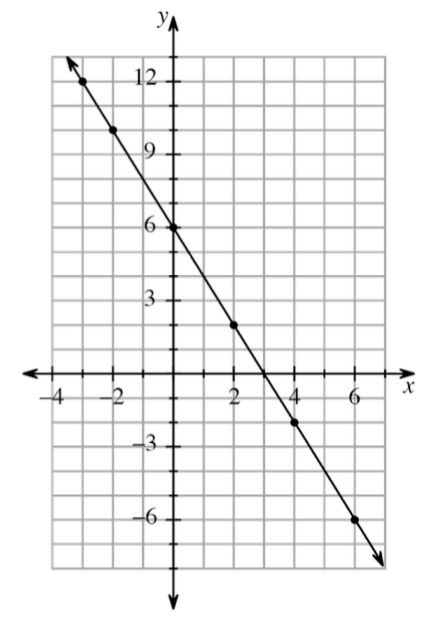### Home > ACC7 > Chapter 6 Unit 7 > Lesson CC3: 6.2.5 > Problem6-96

6-96.

Use the graph below to add points to the table below.

 $x$ x column 1 is blank. x column 2 is blank. x column 3 is blank. x column 4 is blank. x column 5 is blank. x column 6 is blank. x column 7 is blank. $y$ y column 1 is blank. y column 2 is blank. y column 3 is blank. y column 4 is blank. y column 5 is blank. y column 6 is blank. y column 7 is blank.For each point, find its $x$-coordinate and its $y$-coordinate and record the two numbers next to each other in the table.

 $x$ x column 1 $-3$ x column 2 $-2$ x column 3 $0$ x column 4 $2$ x column 5 $4$ x column 6 $6$ $y$ y column 1 $12$ y column 2 $10$ y column 3 $6$ y column 4 $2$ y column 5 $-2$ y column 6 $-6$
1. Write the rule in words.

Notice that for every $1$ the $x$-value increases, the $y$-value decreases by $2$. Also take into account the value for the
$y$-intercept.

Multiply $y$ by $−2$ and then add $6$.

2. Explain how to use the table to predict the value of $y$ when $x$ is $−8$.

Extend the pattern in the table. Answers may vary.